## Example Questions

← Previous 1 3 4

### Example Question #65 : Chemistry

The rate of a reversible chemical reaction depends on many factors, including concentrations of the reactants and products, temperature, and presence of enzymes called catalysts. In the forward reaction, two reactants combine to form one product. However, in a reverse reaction, the product is broken down into the two reactants.

In order for a forward reaction to occur, the reactants moving around in the test tube must physically interact with each other. The more often reactants interact with each other, the more produce is formed in the same amount of time. The speed at which reactants combine into products (the rate of the reaction) can be calculated by dividing the amount of a chemical produced in a reaction (often measured in moles) by the time it takes to produce that amount.

In order to determine the effects of reactant and product concentration, temperature, and presence of catalysts on the rate of a reaction, a scientist studied the following reaction: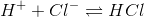The scientist varied the conditions of the experiment and measured the rate of the reaction. The results are outlined in Table 1. The units of concentration are moles per liter.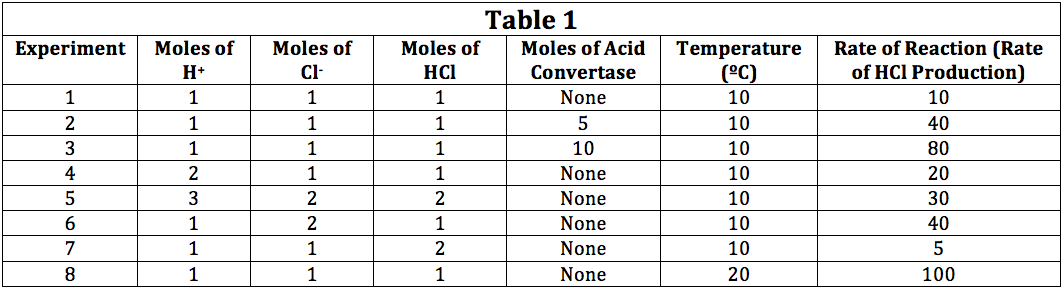In a further experiment, intead of using 5 or 10 moles for the amount of acid covertase, the scientist uses 20 moles. What is the expected rate of reaction?

160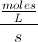1208040160Explanation:

Using Table 1 and Experiments 2 and 3, we can see that doubling the moles of acid convertase doubles the rate. Thus, using 20 moles instead of 10, we would expect to see a double in the rate of 80 to 160 moles per liter per second.

### Example Question #66 : Chemistry

The rate of a reversible chemical reaction depends on many factors, including concentrations of the reactants and products, temperature, and presence of enzymes called catalysts. In the forward reaction, two reactants combine to form one product. However, in a reverse reaction, the product is broken down into the two reactants. If the reverse reaction is running at a quick rate, the overall rate of product formation will be lowered.

In order for a forward reaction to occur, the reactants moving around in the test tube must physically interact with each other. The more often reactants interact with each other, the more produce is formed in the same amount of time. The speed at which reactants combine into products (the rate of the reaction) can be calculated by dividing the amount of a chemical produced in a reaction (often measured in moles) by the time it takes to produce that amount.

In order to determine the effects of reactant and product concentration, temperature, and presence of catalysts on the rate of a reaction, a scientist studied the following reaction:The scientist varied the conditions of the experiment and measured the rate of the reaction. The results are outlined in Table 1. The units of concentration are moles per liter.Why might doubling the number of moles of HCl decrease the rate of HCl production?

The acid convertase enzyme has become inactive

Cannot Be Determined

The acid convertase enzyme is converting HCl back into H+ and Cl-

No more H+ or Cl- exists to be converted

The acid convertase enzyme is converting HCl back into H+ and Cl-

Explanation:

The passage helps us understand that the purpose of an enzyme is to allow for the conversion of reactant to product and product back to reactant. According to Table 1, when the moles of HCl starting the reaction are doubled (Experiments 1 and 7), we see a 50% decrease in the rate of the reaction. It is reasonable that this decrease in the rate of HCl production is due to some of the HCl being driven back to H+ and Cl- according to information contained in the passage, where it says, "if the reverse reaction is running at a quick rate, the overall rate of product formation will be lowered."

### Example Question #61 : Chemistry

The rate of a reversible chemical reaction depends on many factors, including concentrations of the reactants and products, temperature, and presence of enzymes called catalysts. In the forward reaction, two reactants combine to form one product. However, in a reverse reaction, the product is broken down into the two reactants.

In order for a forward reaction to occur, the reactants moving around in the test tube must physically interact with each other. The more often reactants interact with each other, the more produce is formed in the same amount of time. The speed at which reactants combine into products (the rate of the reaction) can be calculated by dividing the amount of a chemical produced in a reaction (often measured in moles) by the time it takes to produce that amount.

In order to determine the effects of reactant and product concentration, temperature, and presence of catalysts on the rate of a reaction, a scientist studied the following reaction:The scientist varied the conditions of the experiment and measured the rate of the reaction. The results are outlined in Table 1. The units of concentration are moles per liter.According to Table 1, how would halving the moles of H+ used change the rate of the reaction?

Decrease by 4 Times

Increase by Two Times

Increase by 4 Times

Decrease by Two Times

Decrease by Two Times

Explanation:

Comparing Experiments 1 and 4 in Table 1, we can see that halving the moles of H+ used in the reaction would drop the rate of the reaction by two times (i.e. halving it).

### Example Question #68 : Chemistry

The rate of a reversible chemical reaction depends on many factors, including concentrations of the reactants and products, temperature, and presence of enzymes called catalysts. In the forward reaction, two reactants combine to form one product. However, in a reverse reaction, the product is broken down into the two reactants.

In order for a forward reaction to occur, the reactants moving around in the test tube must physically interact with each other. The more often reactants interact with each other, the more produce is formed in the same amount of time. The speed at which reactants combine into products (the rate of the reaction) can be calculated by dividing the amount of a chemical produced in a reaction (often measured in moles) by the time it takes to produce that amount.

In order to determine the effects of reactant and product concentration, temperature, and presence of catalysts on the rate of a reaction, a scientist studied the following reaction:The scientist varied the conditions of the experiment and measured the rate of the reaction. The results are outlined in Table 1. The units of concentration are moles per liter.The passage implies that doubling the moles of Cl- used would:

Double the Rate of the Reaction

Triple the Rate of the Reaction

No Change in the Rate of the Reaction

Quadruple the Rate of the Reaction

Quadruple the Rate of the Reaction

Explanation:

Using Table 1 and comparing Experiments 1 and 6, we can see that doubling the moles of Cl- used would quadruple the rate of the reaction from 10 to 40.

### Example Question #1 : How To Find Experimental Design In Chemistry

The rate of a reversible chemical reaction depends on many factors, including concentrations of the reactants and products, temperature, and presence of enzymes called catalysts. In the forward reaction, two reactants combine to form one product. However, in a reverse reaction, the product is broken down into the two reactants.

In order for a forward reaction to occur, the reactants moving around in the test tube must physically interact with each other. The more often reactants interact with each other, the more produce is formed in the same amount of time. The speed at which reactants combine into products (the rate of the reaction) can be calculated by dividing the amount of a chemical produced in a reaction (often measured in moles) by the time it takes to produce that amount.

In order to determine the effects of reactant and product concentration, temperature, and presence of catalysts on the rate of a reaction, a scientist studied the following reaction:The scientist varied the conditions of the experiment and measured the rate of the reaction. The results are outlined in Table 1. The units of concentration are moles per liter.According to Table 1, the function of acid convertase must be to:

Increase the Rate of Reaction

Decrease the Rate of Reaction

Increase the Temperature of the Reaction

Not Enough Information to Determine

Increase the Rate of Reaction

Explanation:

Based on the passage and Table 1, we can see that in Experiments 2 and 3, the increase in acid convertase moles serves to increase the rate of the reaction. Additionally, between Experiments 1 and 2, we see that the addition of acid convertase increases the rate of reaction by four times. Thus, the function of acid convertase must be to increase the rate of the reaction.

### Example Question #70 : Chemistry

The Millikin oil drop experiment is among the most important experiments in the history of science.  It was used to determine one of the fundamental constants of the universe, the charge on the electron. For his work, Robert Millikin won the Nobel Prize in Physics in 1923.

Millikin used an experimental setup as follows in Figure 1. He opened a chamber of oil into an adjacent uniform electric field.  The oil droplets sank into the electric field once the trap door opened, but were then immediately suspended by the forces of electricity present in the field.

Figure 1: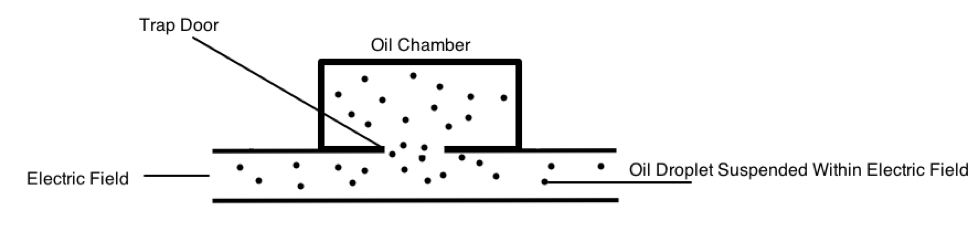By determining how much force was needed to exactly counteract the gravity pulling the oil droplet down, Millikin was able to determine the force of electricity.  This is depicted in Figure 2.

Using this information, he was able to calculate the exact charge on an electron.  By changing some conditions, such as creating a vacuum in the apparatus, the experiment can be modified.

Figure 2: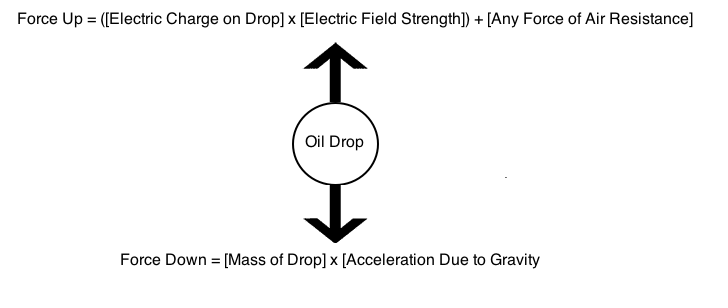When the drop is suspended perfectly, the total forces up equal the total forces down.  Because Millikin knew the electric field in the apparatus, the force of air resistance, the mass of the drop, and the acceleration due to gravity, he was able to solve the following equation: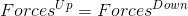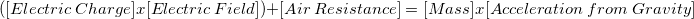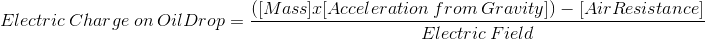Table 1 summarizes the electric charge found on oil drops in suspension.  Millikin correctly concluded that the calculated charges must all be multiples of the fundamental charge of the electron.  A hypothetical oil drop contains some net charge due to lost electrons, and this net charge cannot be smaller than the charge on a single electron.

Table 1:

 Trial # Electric Charge Calculated in Coulombs (C) Vacuum Used? 1 1.602176487 x 10-8 No 2 1.602176487 x 10-2 Yes 3 1.602176487 x 10-6 No 4 1.602176487 x 10-4 Yes

The electric force experienced by oil drops will vary directly with the magnitude of charge on the drop.  A scientist is measuring two different drops in two different experimental apparatuses, but each in perfect suspension and not moving.  Drop 1 has a greater net charge than does drop 2.  The magnitude of the net total force:

May be greater on either drop 1 or drop 2

Is greater on drop 1 than drop 2

Is greater on drop 2 than drop 1

Is initially greater on drop 1, but then is greater on drop 2

Is the same on both drops

Is the same on both drops

Explanation:

The question specifies that both drops are suspended and not moving.  Thus, the net force on both drops must be the same.

### Example Question #1 : How To Find Experimental Design In Chemistry

A researcher studies the olfactory (scent-related) senses of the giant forest ant, Camponotus gigas. The researcher places 120 ants in a three-chambered cell. The cell has an end section with a cotton ball soaked in a saline solution and another end with a cotton ball soaked in a glucose solution. The ants are placed in the middle and timed for 15 minutes. Their initial and final positions in the cell are recorded (see Table 1). The researcher's null hypothesis states that the distribution of ants across the three chambers will be equal to one another. In other words, the glucose solution will have no effect and there will be no significant difference in the distribution of the insects.

Table 1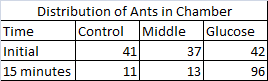The researcher decides to perform a statistical test known as a Chi-squared test of independence to interpret the experiment's results. The test is performed by calculating a Chi-squared statistic by utilizing observed and expected values for distribution (see Table 2). If the sum of the Chi-squared test statistic is higher than the critical value, then the null hypothesis can be rejected. This indicates that the distribution of insects is not random and the variable in question has a pronounced effect on the subjects. A critical value is calculated by determining the degrees of freedom, which in this experiment is equal to the number of categories in the study minus one, and then locating the proper number on a table (see Table 3). There are three possible categories in this experiment: the glucose end, the control end (with the saline-solution soaked cotton ball), and middle portion of the chamber.

Table 2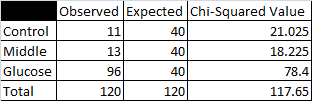Table 3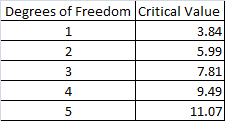What is the target organism of this study?

Tapinoma melanocephalum

Harpegnathos saltator

Protomognathus americanus

Camponotus gigas

Camponotus gigas

Explanation:

The first paragraph of the passage states that the target organism of the study is the ant species Camponotus gigas. The researcher was attempting to study the olfactory senses of the ants in response to a glucose food source. This is supported by the passage.

### Example Question #2 : How To Find Experimental Design In Chemistry

During digestion, the energy in food is converted to energy the body can use. Scientists use calorimetry experiments to measure the calories, or energy, provided by food when it is digested or burned.

The relationship used to find the heat transferred energy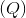is given by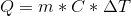, whereis the mass of the material,is the given specific heat capacity, and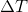is the change in temperature of the material.

In this experiment, food was burned over a Bunsen burner under a can of 200 ml of water. The temperature change of the water and mass change of the food can be used to determine the calories in four different food items.

Table 1 shows the values of the change of mass of the food items, the change in temperature of the water and the energy. Table 2 shows the energy to mass ratio of three of those food items.

Table 1

Roasted Peanut  Peanut    Cracker    Cheese Puff

Water Temp. Initial         23.9 °C           33.2 °C     40.3 °C      53.9 °C

Water Temp. Final           30.0 °C           40.9 °C     55.9 °C      62.8 °C

Food Mass Initial             0.69 g             0.61 g       3.21 g        1.22 g

Food Mass Final               0.38 g             0.21 g       0.91 g        0.48 g

Energy                            1.22 Cal          1.54 Cal     3.12 Cal     1.78 Cal

Table 2

Sample         Energy to Mass Ratio (Cal/g)

1                                 1.36

2                                 3.93

3                                 2.40

What is a controlled variable in this experiment?

The initial mass of the food items

The specific heat capacity of water

The initial temperature of the water

The specific heat capacity of the food items

The specific heat capacity of water

Explanation:

The specific heat capacity of the water is the only constant out of this group because the same water is used throughout the four samples. All of the other conditions are shown to change based on the values in Table 1. Additionally, maintaining a constant specific heat capacity in the water is essential for finding the calories in the food though this method.

### Example Question #3 : How To Find Experimental Design In Chemistry

During digestion, the energy in food is converted to energy the body can use. Scientists use calorimetry experiments to measure the calories, or energy, provided by food when it is digested or burned.

The relationship used to find the heat transferred energyis given by, whereis the mass of the material,is the given specific heat capacity, andis the change in temperature of the material.

In this experiment, food was burned over a Bunsen burner under a can of 200 ml of water. The temperature change of the water and mass change of the food can be used to determine the calories in four different food items.

Table 1 shows the values of the change of mass of the food items, the change in temperature of the water and the energy. Table 2 shows the energy to mass ratio of three of those food items.

Table 1

Roasted Peanut  Peanut    Cracker    Cheese Puff

Water Temp. Initial         23.9 °C           33.2 °C     40.3 °C      53.9 °C

Water Temp. Final           30.0 °C           40.9 °C     55.9 °C      62.8 °C

Food Mass Initial             0.69 g             0.61 g       3.21 g        1.22 g

Food Mass Final               0.38 g             0.21 g       0.91 g        0.48 g

Energy                            1.22 Cal          1.54 Cal     3.12 Cal     1.78 Cal

Table 2

Sample         Energy to Mass Ratio (Cal/g)

1                                 1.36

2                                 3.93

3                                 2.40

Based on the information in Table 1, what variables must be measured in order to calculate the energy of the food samples?

Change in mass of the food and change in temperature of the water

Change in mass of the food sample alone

Change in mass and temperature of the water

Change in temperature of the water alone

Change in mass of the food and change in temperature of the water

Explanation:

Based on the given equation for calculating heat energy, the heat capacity of the water, change of mass of the material, and change in temperature of the material must be measured. However, because the heat capacity of water is consistent, it does not affect the measure of the different calorie measurements. Additionally, because the change in heat of the food samples must approximately equal the change in temperature of the food item due to their proximity, only the change in temperature of the water and change in mass of the food samples needs to be known. Also, this answer is given in the description of the experiment.

### Example Question #4 : How To Find Experimental Design In Chemistry

A student wants to perform an experiment that tests the relationship between the pressure of a gas and the volume it occupies. To perform this experiment, the student places a specific type of gas in a sealed chamber that can change pressure and that can adapt its volume to the gas within it. The chamber also adjusts to the changing pressure such that the temperature (which also has an effect on gas volume) does not change. The following data was obtained: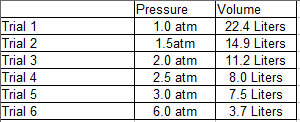Based on the information in the passage, which variable(s) are or were held as constants?

Temperature and type of gas

Size of chamber

Temperature, type of gas, and size of chamber

Temperature

Type of gas